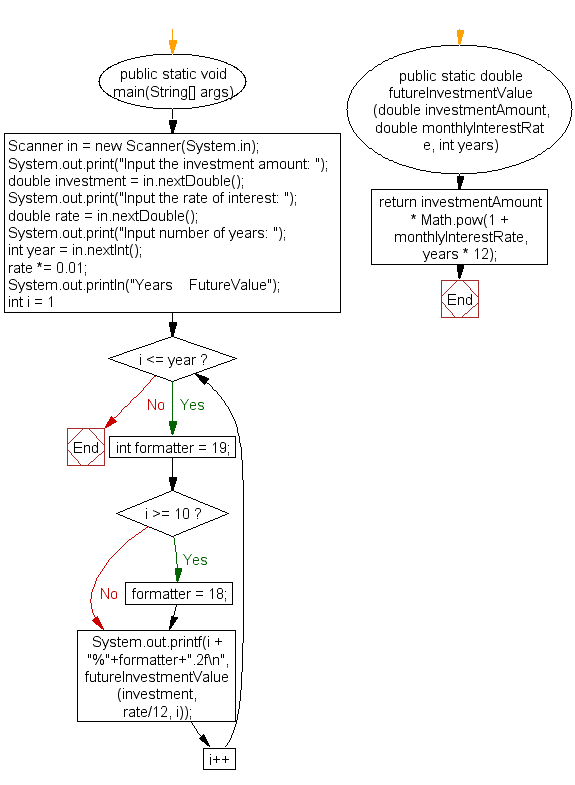﻿ Java exercises: Compute the future investment value at a given interest rate for a specified number of years - w3resource# Java Method Exercises: Compute the future investment value at a given interest rate for a specified number of years

## Java Method: Exercise-8 with Solution

Write a Java method to compute the future investment value at a given interest rate for a specified number of years.

Sample data (Monthly compounded):
Input the investment amount: 1000
Input the rate of interest: 10
Input number of years: 5

Sample Solution:

Java Code:

``````import java.util.Scanner;
public class Exercise8 {

public static void main(String[] args) {
Scanner in = new Scanner(System.in);
System.out.print("Input the investment amount: ");
double investment = in.nextDouble();
System.out.print("Input the rate of interest: ");
double rate = in.nextDouble();
System.out.print("Input number of years: ");
int year = in.nextInt();

rate *= 0.01;

System.out.println("Years    FutureValue");
for(int i = 1; i <= year; i++) {
int formatter = 19;
if (i >= 10) formatter = 18;
System.out.printf(i + "%"+formatter+".2f\n", futureInvestmentValue(investment, rate/12, i));
}
}

public static double futureInvestmentValue(double investmentAmount, double monthlyInterestRate, int years) {
return investmentAmount * Math.pow(1 + monthlyInterestRate, years * 12);
}
}
```
```

Sample Output:

```Input the investment amount: 1000
Input the rate of interest: 10
Input number of years: 5
Years    FutureValue
1            1104.71
2            1220.39
3            1348.18
4            1489.35
5            1645.31
```

Flowchart:Java Code Editor:

What is the difficulty level of this exercise?

﻿

## Java: Tips of the Day

Array vs ArrayLists:

The main difference between these two is that an Array is of fixed size so once you have created an Array you cannot change it but the ArrayList is not of fixed size. You can create instances of ArrayLists without specifying its size. So if you create such instances of an ArrayList without specifying its size Java will create an instance of an ArrayList of default size.

Once an ArrayList is full it re-sizes itself. In fact, an ArrayList is internally supported by an array. So when an ArrayList is resized it will slow down its performance a bit as the contents of the old Array must be copied to a new Array.

At the same time, it's compulsory to specify the size of an Array directly or indirectly while creating it. And also Arrays can store both primitives and objects while ArrayLists only can store objects.

Ref: https://bit.ly/3o8L2KH